# F.E.A.R. 3 Walkthrough Interval 02: Slums

September 12, 2016 (Last Updated: ) | Reading Time: 3 minutes

This F.E.A.R. 3 walkthrough is divided into 8 total pages.

2 - Interval 02: Slums

You can jump to nearby pages of the game using the links above.

## Interval 02: Slums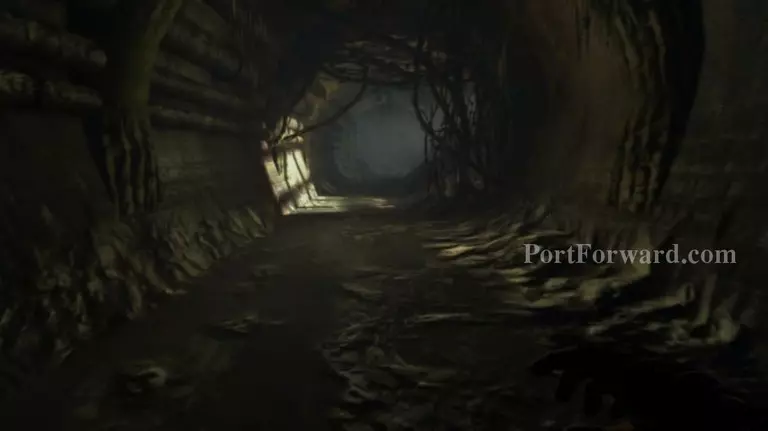F.E.A.R. 3 Walkthrough - F.E.A.R. 3 41

Get to the end of the tunnel.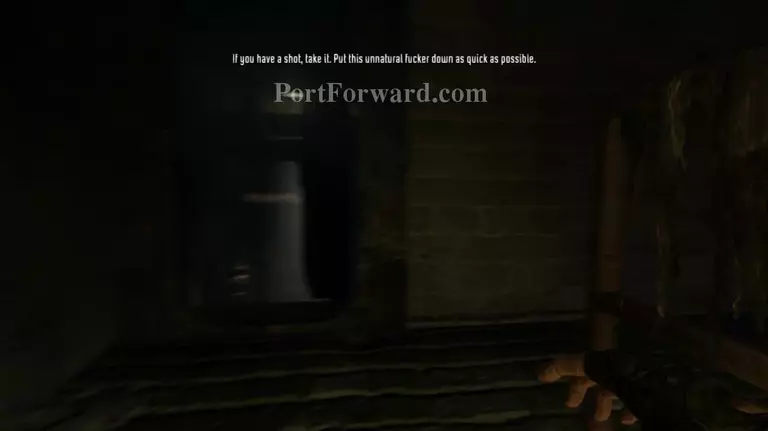F.E.A.R. 3 Walkthrough - F.E.A.R. 3 42

Go through the door.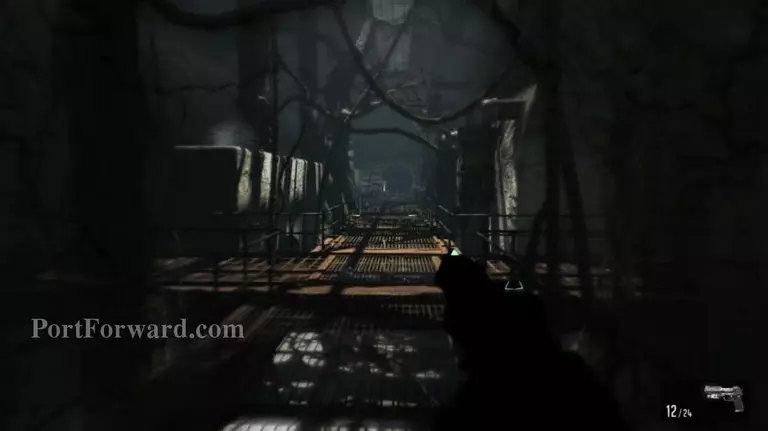F.E.A.R. 3 Walkthrough - F.E.A.R. 3 43

Get to the other end of the walkway.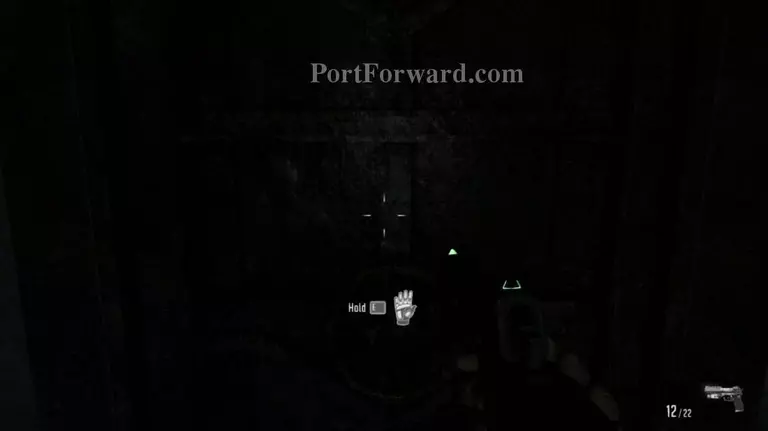F.E.A.R. 3 Walkthrough - F.E.A.R. 3 44

Open the door.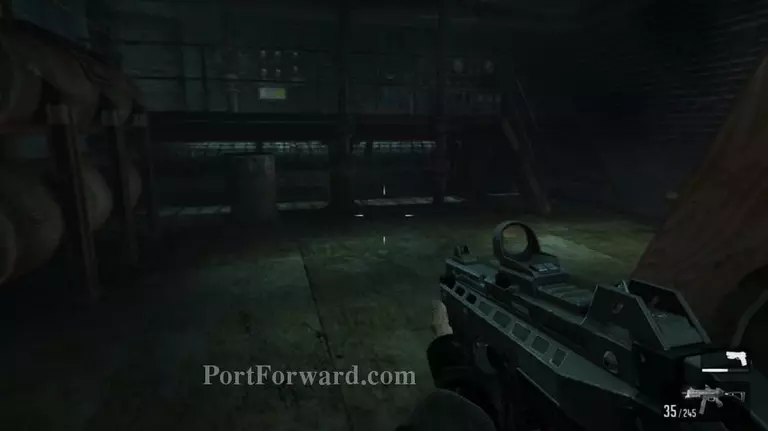F.E.A.R. 3 Walkthrough - F.E.A.R. 3 45

Keep going forward.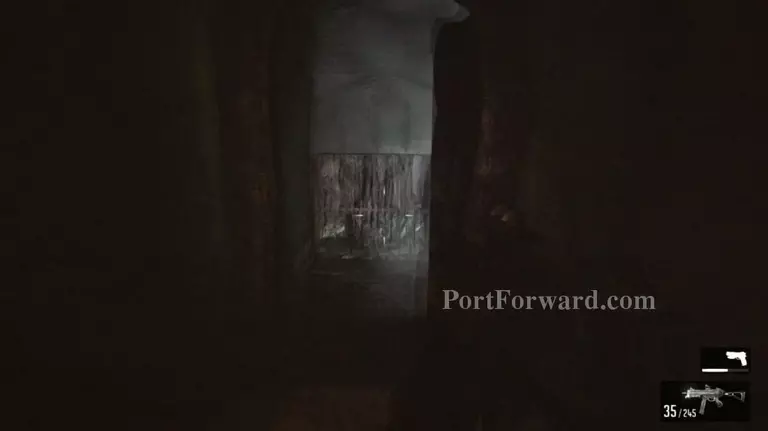F.E.A.R. 3 Walkthrough - F.E.A.R. 3 46

Jump over the railing and follow the tunnel.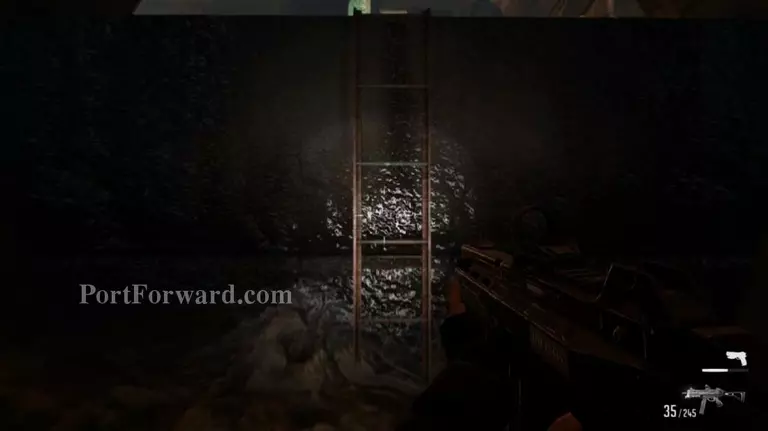F.E.A.R. 3 Walkthrough - F.E.A.R. 3 47

Go up the ladder.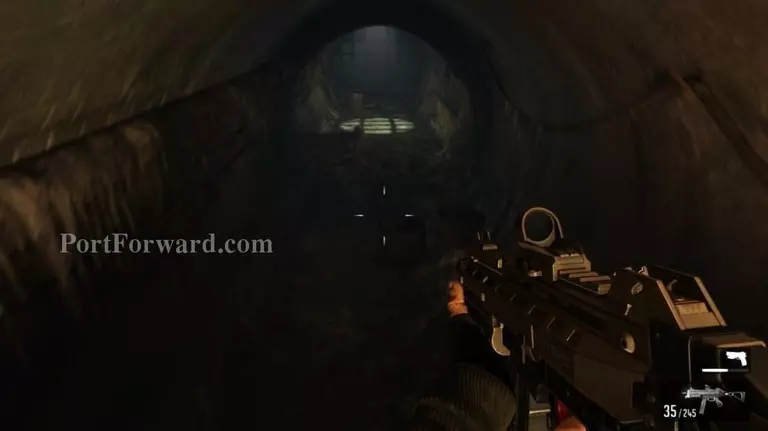F.E.A.R. 3 Walkthrough - F.E.A.R. 3 48

Jump down into the other tunnel and follow the path.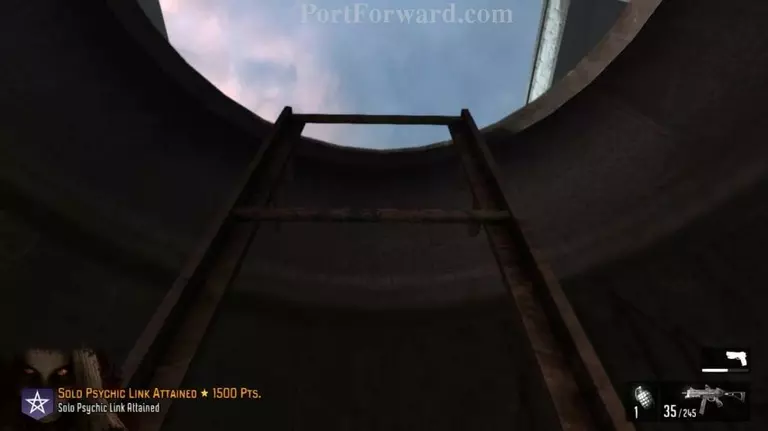F.E.A.R. 3 Walkthrough - F.E.A.R. 3 49

Up another ladder. There'll be guards ahead.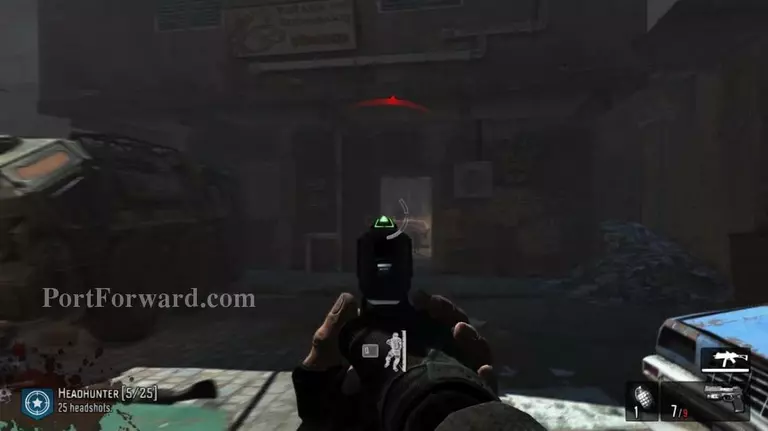F.E.A.R. 3 Walkthrough - F.E.A.R. 3 50

Make your way into the house at the end of the street.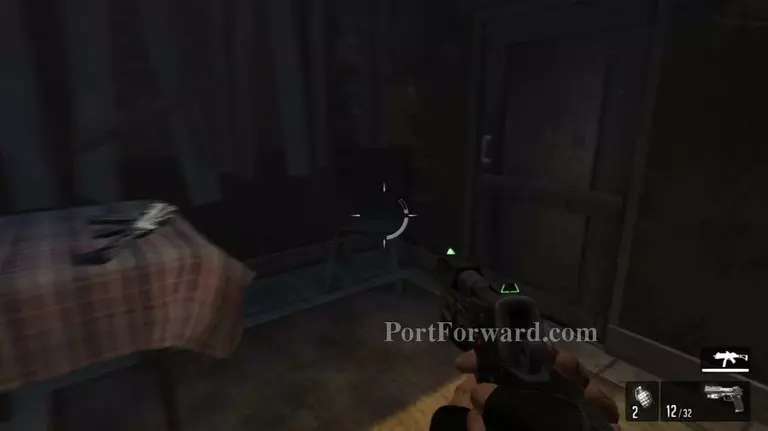F.E.A.R. 3 Walkthrough - F.E.A.R. 3 51

Proceed through the door inside the house.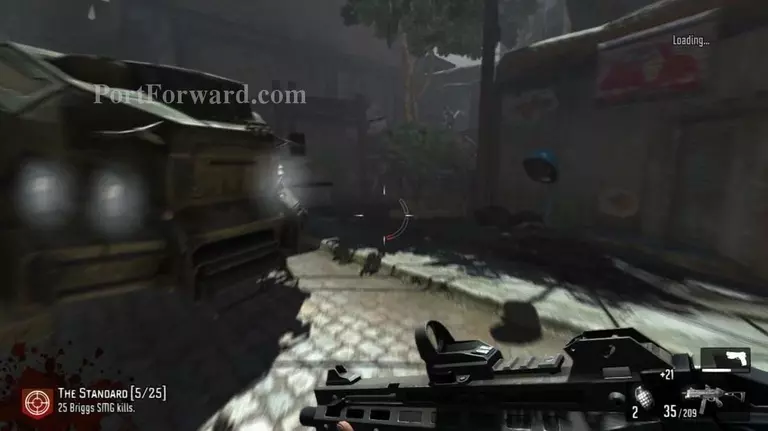F.E.A.R. 3 Walkthrough - F.E.A.R. 3 52

Take the bad guys out, then go up the staircase to the side of the truck.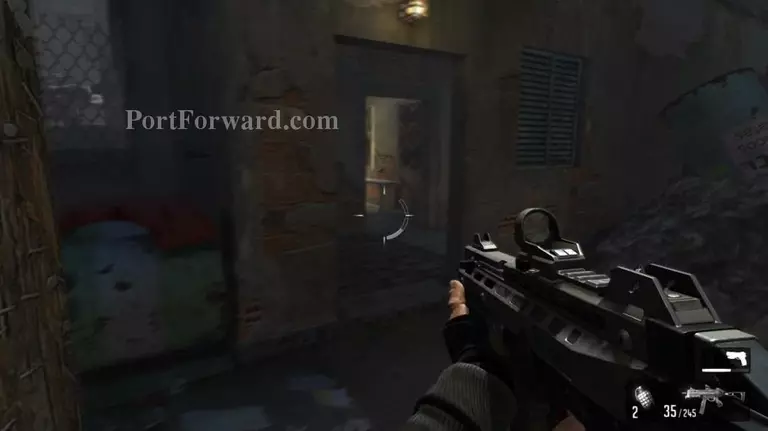F.E.A.R. 3 Walkthrough - F.E.A.R. 3 53

Continue through the house.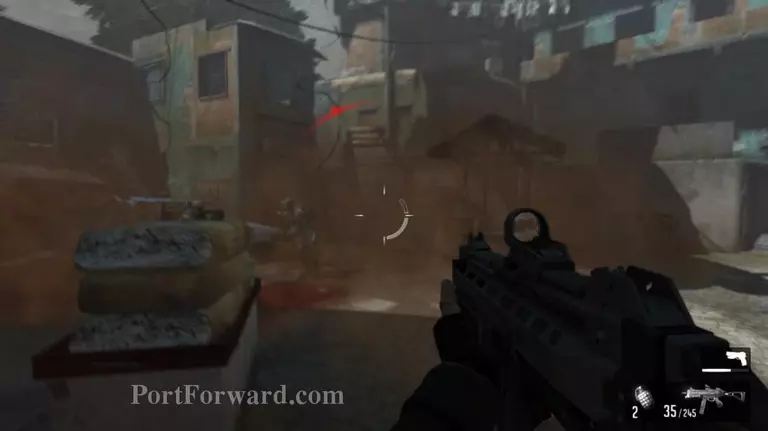F.E.A.R. 3 Walkthrough - F.E.A.R. 3 54

Outside there'll be plenty of enemies. Get ready.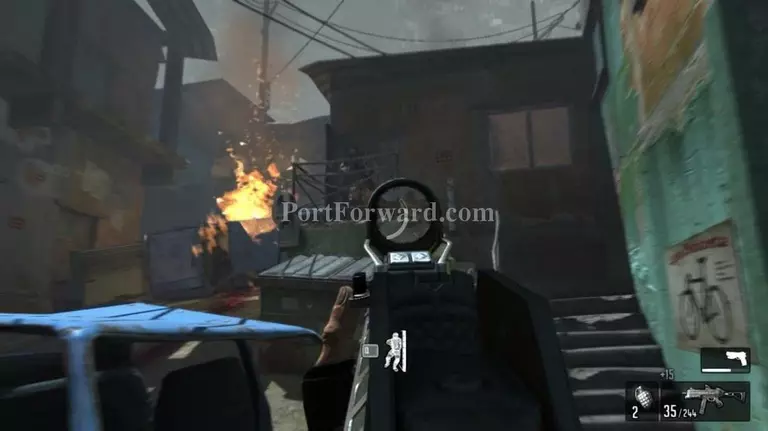F.E.A.R. 3 Walkthrough - F.E.A.R. 3 55

Kill all the soldiers in the area. The last one will come busting the door open at the top of the staircase. Get rid of him then go inside.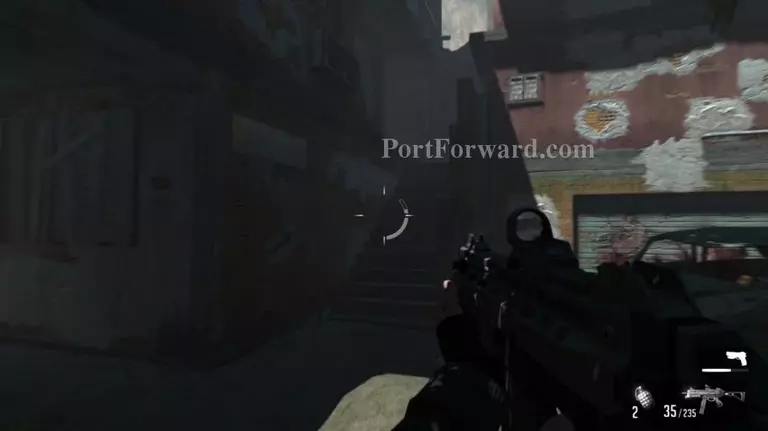F.E.A.R. 3 Walkthrough - F.E.A.R. 3 56

Go out the door and up the stairs in front.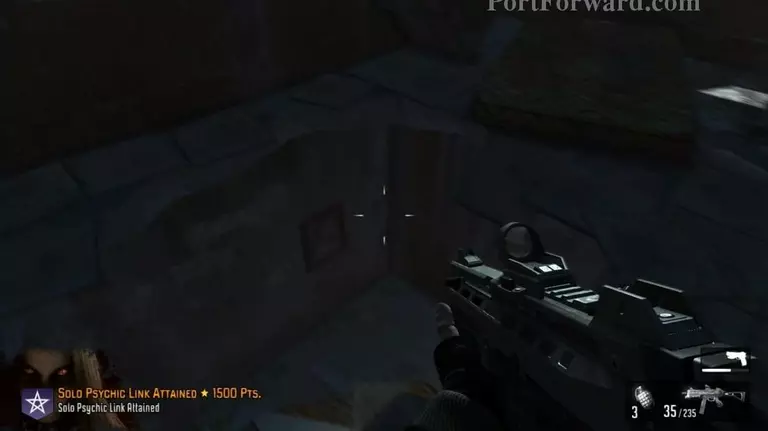F.E.A.R. 3 Walkthrough - F.E.A.R. 3 57

Drop down the hole on the floor.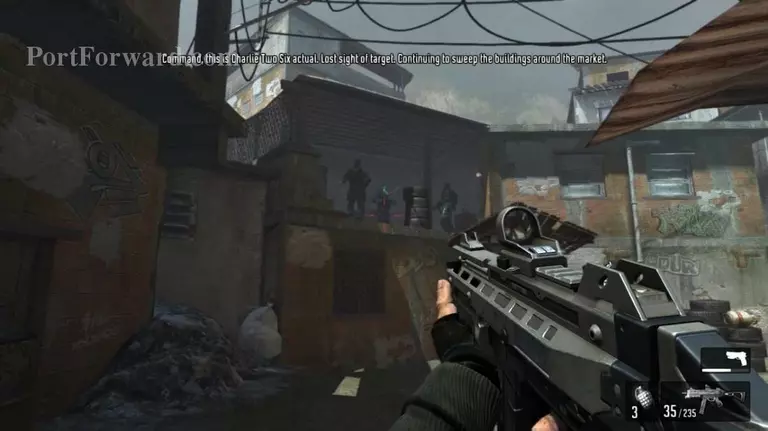F.E.A.R. 3 Walkthrough - F.E.A.R. 3 58

You'll find more bad guys outside.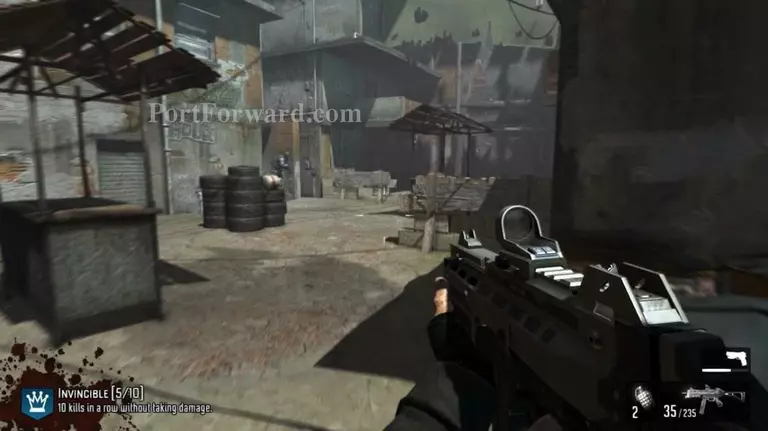F.E.A.R. 3 Walkthrough - F.E.A.R. 3 59

Make your way forward.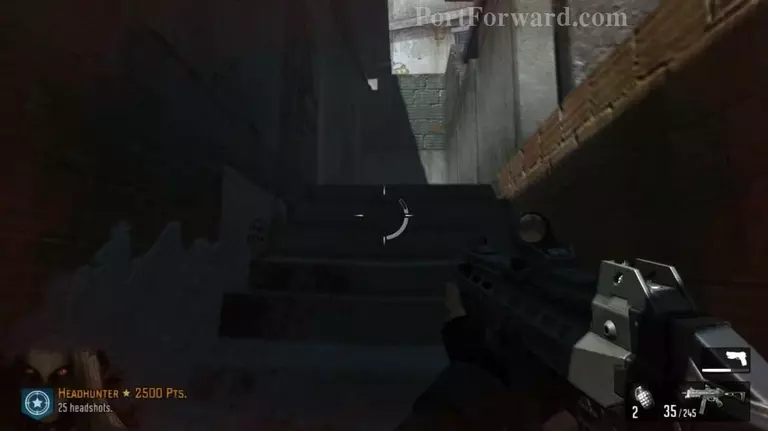F.E.A.R. 3 Walkthrough - F.E.A.R. 3 60

Go upstairs and deal with the remaining guards.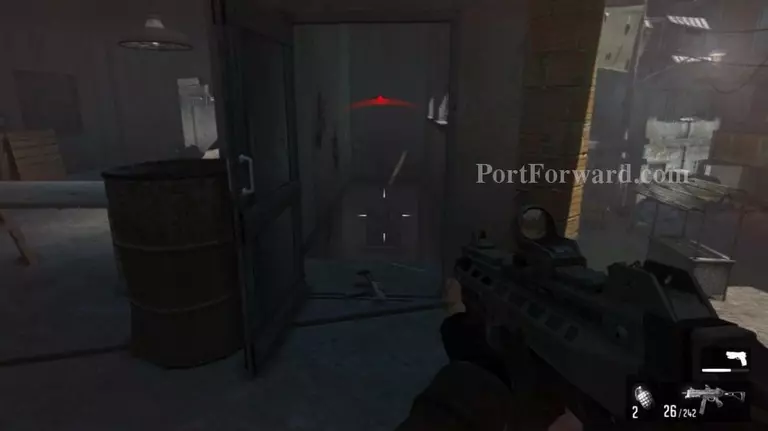F.E.A.R. 3 Walkthrough - F.E.A.R. 3 61

Go through the door the guard opened.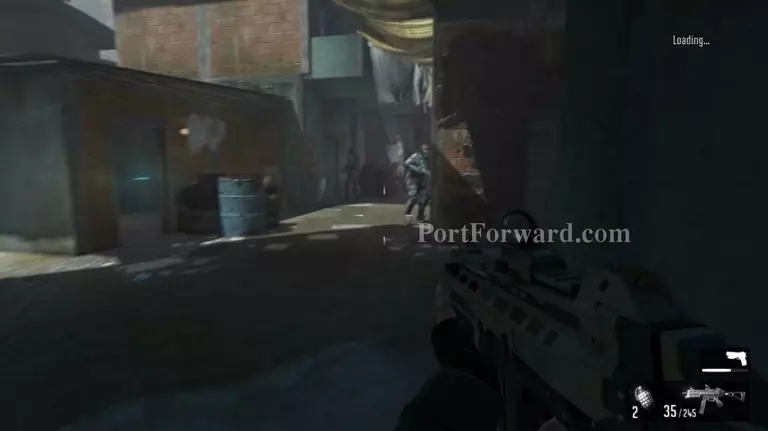F.E.A.R. 3 Walkthrough - F.E.A.R. 3 62

More guards at the end of the path.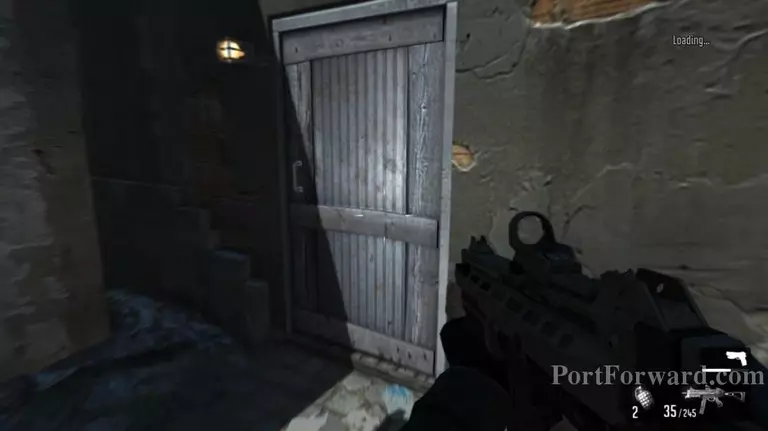F.E.A.R. 3 Walkthrough - F.E.A.R. 3 63

Into the door at the end of the street.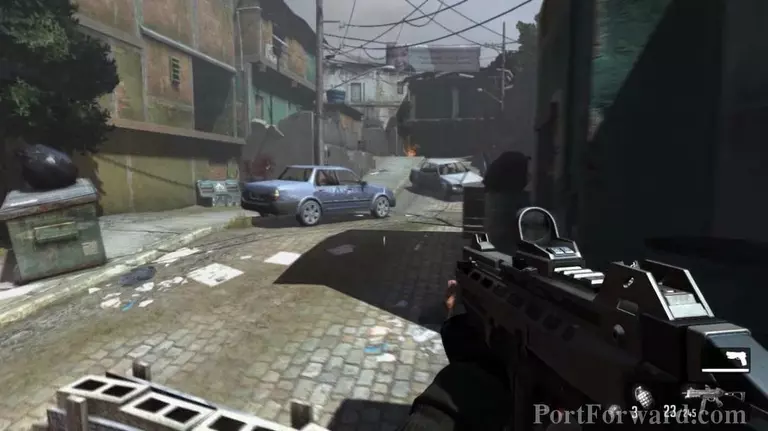F.E.A.R. 3 Walkthrough - F.E.A.R. 3 64

Ahead you'll find guards and a mech.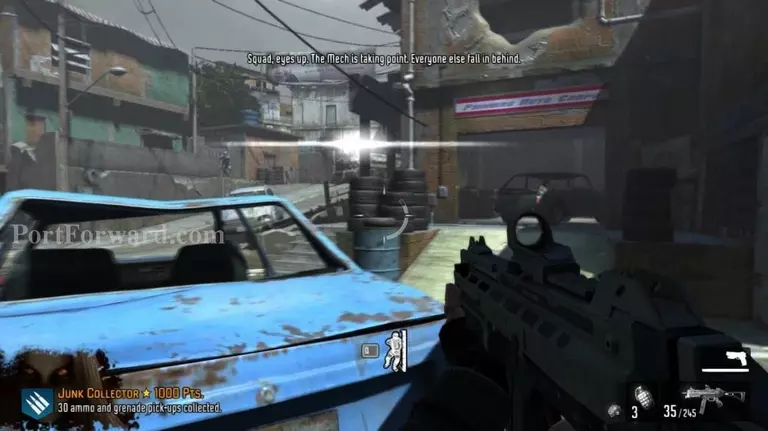F.E.A.R. 3 Walkthrough - F.E.A.R. 3 65

Go into the house on the right and up to second floor.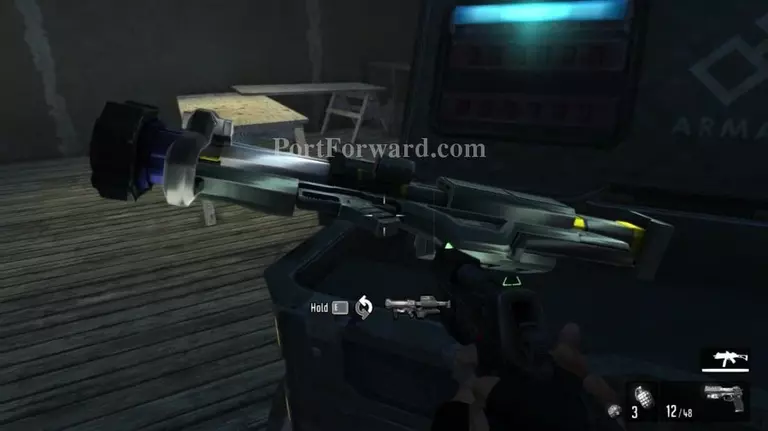F.E.A.R. 3 Walkthrough - F.E.A.R. 3 66

Grab the rocket launcher.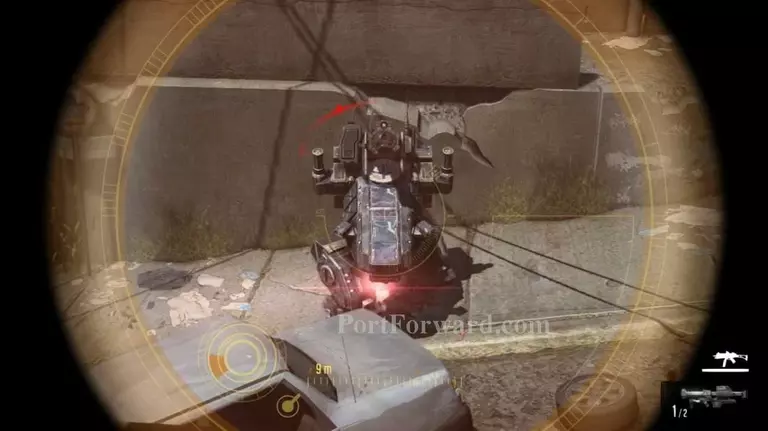F.E.A.R. 3 Walkthrough - F.E.A.R. 3 67

Destroy the mech.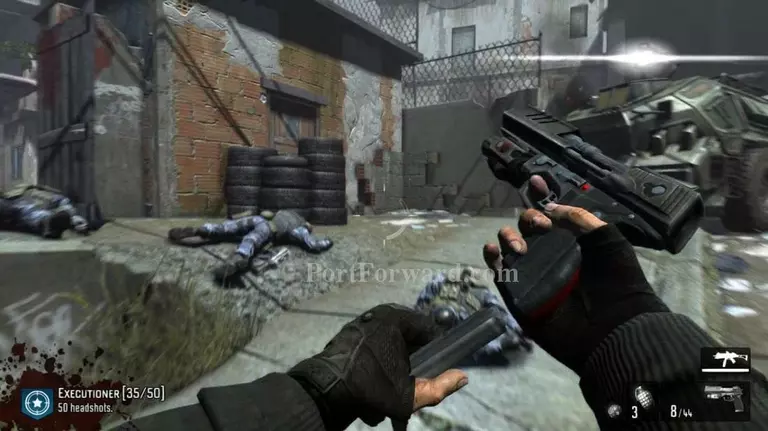F.E.A.R. 3 Walkthrough - F.E.A.R. 3 68

Go into the alley to the left of the truck.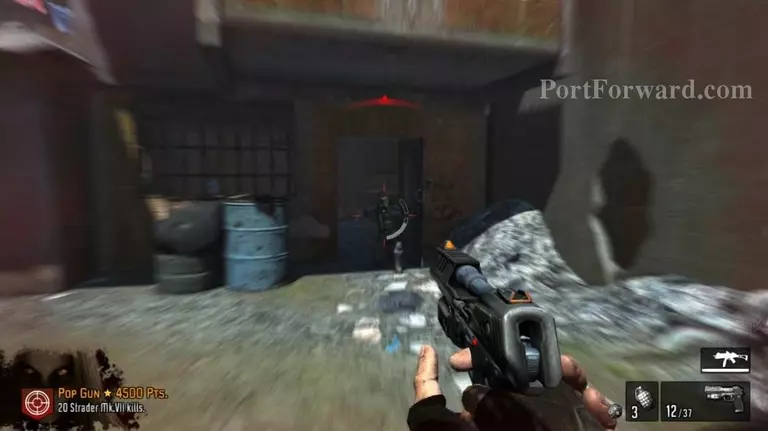F.E.A.R. 3 Walkthrough - F.E.A.R. 3 69

Kill the soldiers then head left.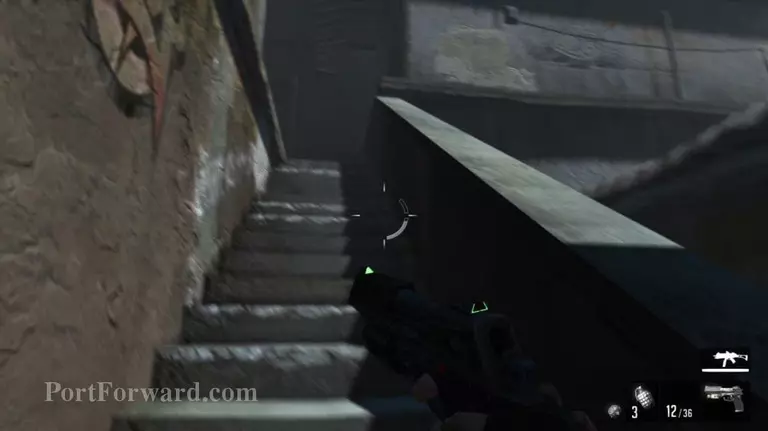F.E.A.R. 3 Walkthrough - F.E.A.R. 3 70

Go up the stairs.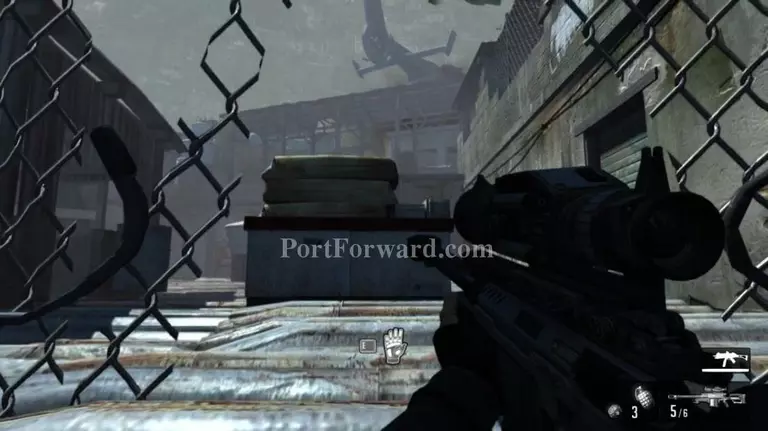F.E.A.R. 3 Walkthrough - F.E.A.R. 3 71

Go through the fence.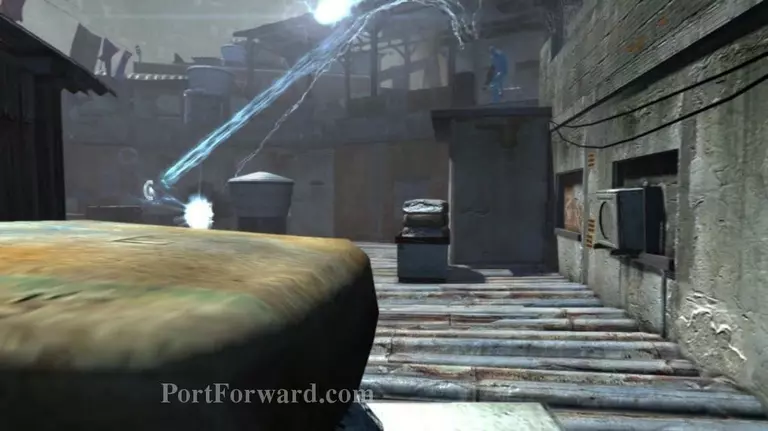F.E.A.R. 3 Walkthrough - F.E.A.R. 3 72

Watch out for the blue guy as he can spawn enemies and has a laser gun. Aim for his head.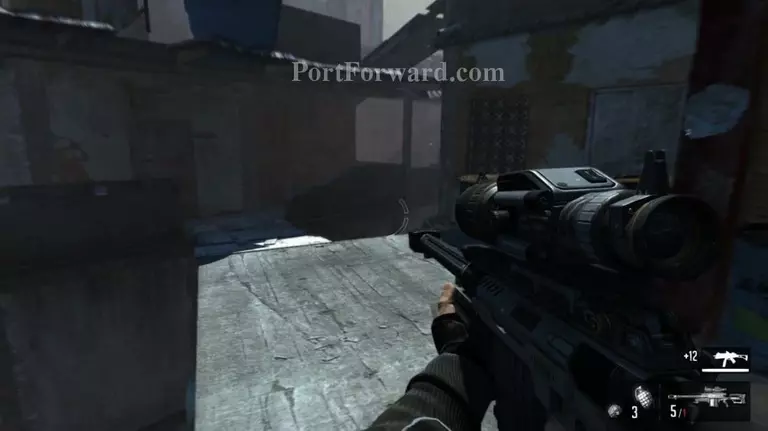F.E.A.R. 3 Walkthrough - F.E.A.R. 3 73

Fight your way up from rooftop to rooftop.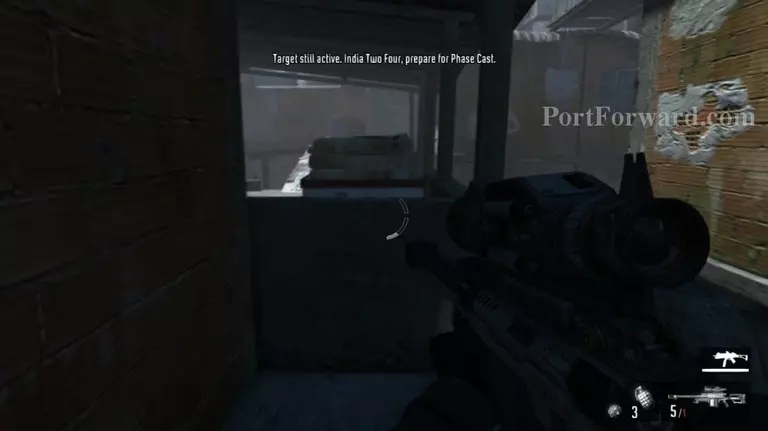F.E.A.R. 3 Walkthrough - F.E.A.R. 3 74

Vault over the low wall.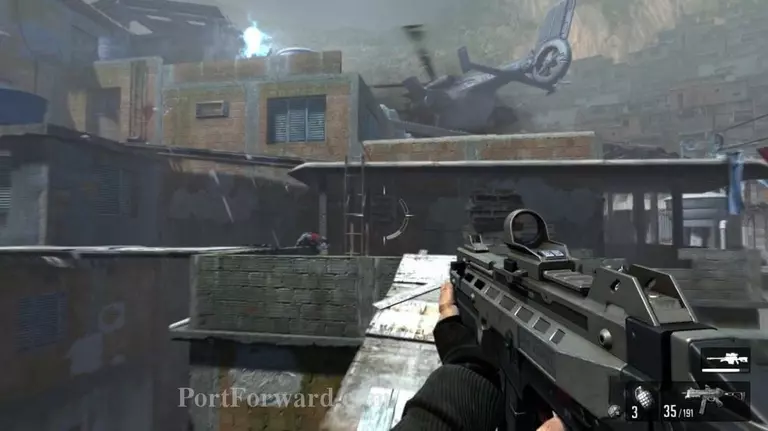F.E.A.R. 3 Walkthrough - F.E.A.R. 3 75

Walk across and go up the ladder.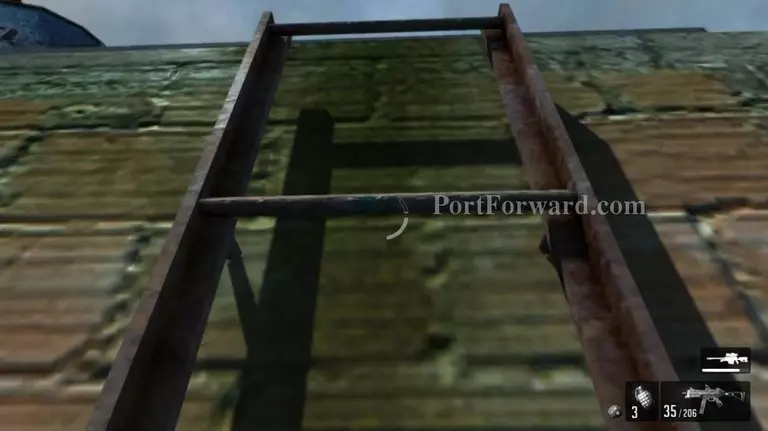F.E.A.R. 3 Walkthrough - F.E.A.R. 3 76

Up the second ladder.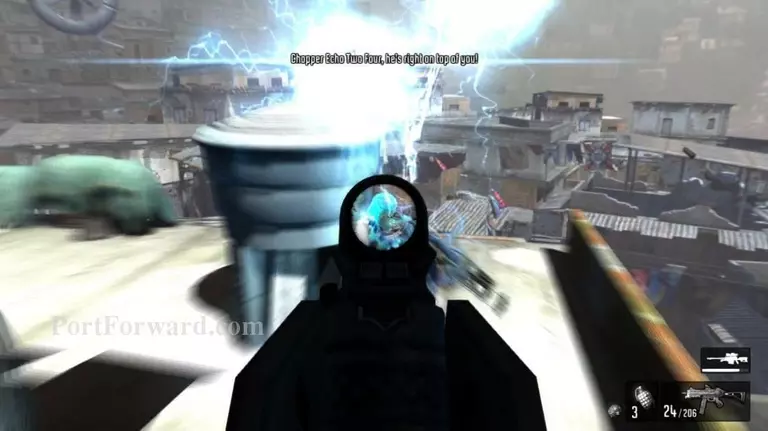F.E.A.R. 3 Walkthrough - F.E.A.R. 3 77

Finish off the enemies left.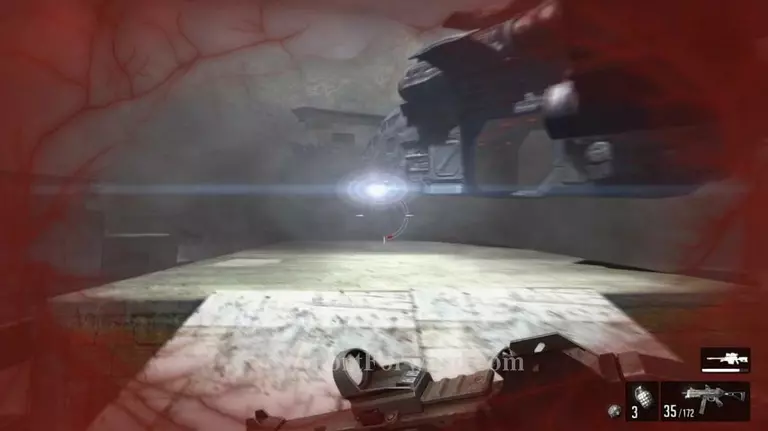F.E.A.R. 3 Walkthrough - F.E.A.R. 3 78

Get in the helicopter.

## More F.E.A.R. 3 Walkthroughs

This F.E.A.R. 3 walkthrough is divided into 8 total pages.

2 - Interval 02: Slums